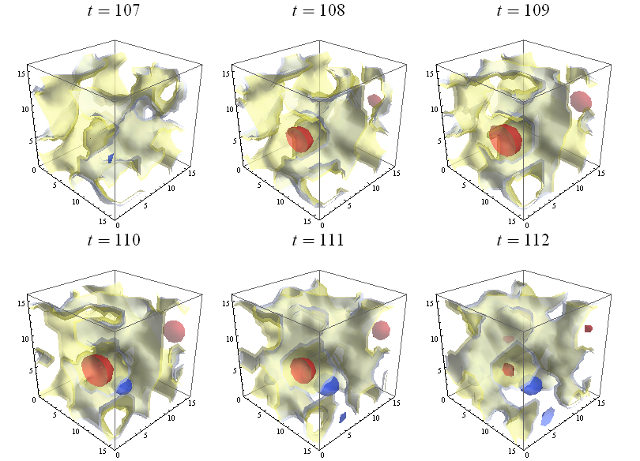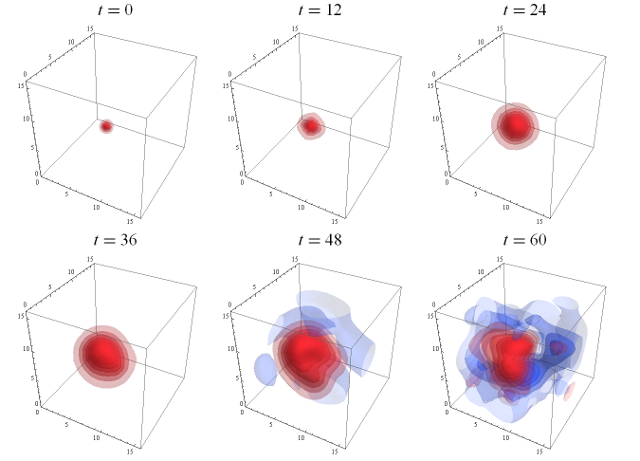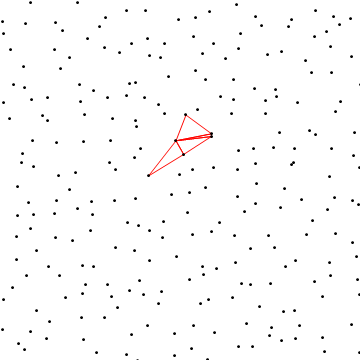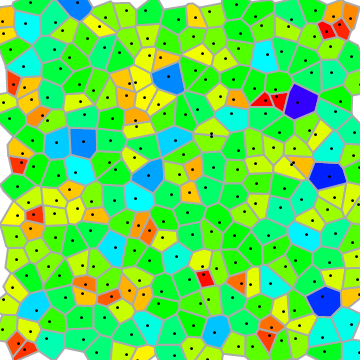## Research Topics

My research focuses on using high-performance supercomputers to nonperturbatively calculate physical quantities at the quark and gluon level (that is, using quantum chromodynamics or QCD). These strong interactions are directly calculated from the Standard Model path integral, using a four-dimensional grid in Euclidean spacetime, a theoretical tool known as lattice gauge theory. Using lattice QCD, I am investigating particle properties that will help discern the difference between new physics and the Standard Model in searches at the LHC and beyond. I am working to overcome wide-ranging challenges that face the lattice community, in particular, deriving quantities with applications to new-physics searches at the intensity frontier.

• Online lecture videos from the lattice-QCD summer school I organized at INT in 2012
• "Lattice Methods for Quantum Chromodynamics", Degrand and De Tar, World Scientific, 2006.
• "Introduction to Lattice QCD", Gupta, arXiv:hep-lat/9807028.

## Quantum chromodynamics (QCD) and Lattice Gauge Theory

My main research so far has been focused on applications of QCD. It is well known as the theory describing the strong interactions of fundamental particles. When the coupling becomes weak at short distances, we can perform perturbative calculations to extract physics analytically. For the past three decades, physicists have devoted their time to studying the phenomena of this theory that can be well described perturbatively (such as in homework problems in the graduate field theory classes). However, we also know that QCD differs from QED because of asymptotic freedom (this was the 2004 Nobel Prize for Physics); the coupling constants becomes too big the usual perturbation calculations to converge. A straightforward solution for dealing with phenomena in such a nonperturbative region would be to use lattice gauge theory. This is the primary focus of my research.

Lattice QCD is a discrete version of the continuum QCD theory. The path integral over field strengths at infinitely many Minkowski space-time points from continuum QCD is approximated using only finitely many points in a discrete Euclidian space lattice. In momentum-space, this automatically provides both an ultraviolet cutoff at the inverse lattice spacing and an infrared cutoff at the inverse box size. However, the breaking of continuous (Euclidean) SO(4) symmetry allows many new degrees of freedom, leading to more complicated lattice actions and operators (and operator mixing problems). This can be systematically tracked and kept under control step-by-step, and one can put the path intergral of the desired physics quantities on a computer to get QCD.

Here are some examples of lattice gauge theory calculations:A selection of time-slices showing the topological charge of the vacuum calculated on one gauge-field configuration on a lattice with spacing approximately 0.1227 × 10−15 meters with 16 lattice sites in each spatial direction. The evolution of the vacuum shown in this image occurs over 5.8 × 10−25 seconds. The red regions correspond to instantons with positive topological winding number, while the blue correspond to instantons with negative winding number.A selection of time-slices showing the real part of the proton correlation function calculated on one gauge-field configuration on a lattice with spacing approximately 0.1227 × 10−15 meters, having 16 lattice sites in each spatial direction. The difference between adjacent time-slices corresponds to Δt = 1.17 × 10−25 seconds, and the evolution of the proton correlation function shown in this image occurs over 7.02 × 10−24 seconds. To enhance detail, the colors (red being more positive and blue more negative) have been normalized on each time-slice. The proton source on time-slice t = 0 has a Gaussian distribution in space.Transverse charge densities in an polarized proton (left) and neutron (right) in the two-dimensional impact plane. To obtain these plots, we first calculate nucleon Dirac and Pauli form factors and then Fourier transform them (with some Bessel-function weight) into a description of the spatial structure of the nucleon. In the figures, black indicates near-zero values, and purple, orange and white are increasingly positive. For the right figure, the same color-scale applies, slightly negative values are gold and the most negative are green.Two-dimensional random lattice (16 × 16). (Left) The construction begins by randomly selecting points from a uniform interval in each dimension; the color of lines indicates the ordering of the construction. (Right) Voronoi tessellation of the same lattice. A division of space into cells, each of which contains all parts of the space closer to its associated point than to any other point. Color indicates the size of the cell (blue is largest, red smallest).